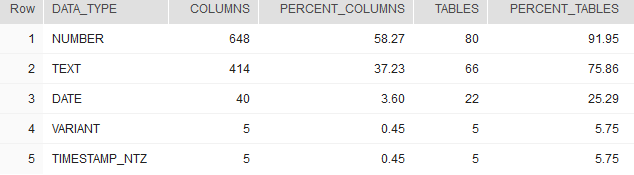## Find most used data type in Snowflake

Article for:

Query below returns data types used in a database ordered by the number of their occurance.

## Query

``````select  c.data_type,
count(*) as columns,
cast(100.0 *
count(*) /
(select count(*) from information_schema.tables t
inner join information_schema.columns c on
c.table_schema = t.table_schema
and c.table_name = t.table_name
where table_type = 'BASE TABLE')
as decimal (14,2))  as percent_columns,
count(distinct t.table_schema || '.' ||  t.table_name ) as tables,
cast(100.0 *
count(distinct t.table_schema || '.' ||  t.table_name) /
(select count(*) from information_schema.tables
where table_type = 'BASE TABLE')
as decimal (14,2)) as percent_tables
from information_schema.tables t
inner join information_schema.columns c on
c.table_schema = t.table_schema and c.table_name = t.table_name
where table_type = 'BASE TABLE'
group by c.data_type
order by count(*) desc;
``````

## Columns

• data_type - built in or user data type without length or precision, e.g. int, varchar or date
• columns - number of columns in a database with this data type
• percent_columns - percentage of columns with this data type. Rows add up to 100%
• tables - number of tables in a database with this data type
• percent_tables - percentage of tables with columns with this data type.

## Rows

• One row represents one data type
• Scope of rows: all data types used in a database
• Ordered by number of columns descending

## Sample results

Those results show data types used in SNOWFLAKE_SAMPLE_DATA database. As you can see most popular data type is number - it is used in 648 columns which is 58.27% of all columns in 80 tables (which 91.95% of all tables).